# Understanding Loop And Conditional Structures in Onboard Programming

Publish Date: Sep 02, 2016 | 12 Ratings | 2.67 out of 5 | Print

You can create loops in onboard programs by using the ‘Insert Label’ function with the ‘Jump To Label On Condition’ function. This is somewhat similar to how you create loops in BASIC with line numbers and GOTO statements. In an onboard program, you can insert a label somewhere in the program and when the ‘Jump To Label On Condition’ is called, if the condition is met, the program will go to where the label is.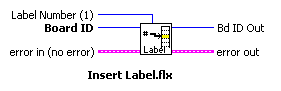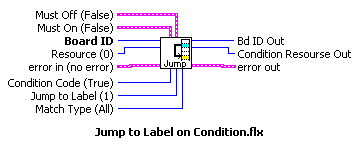The most common loops that you can create on onboard programming are FOR loops and DO-WHILE loops and we will also discuss the conditional structure IF-ELSE. These structures (loop and conditional) are included as example programs in the shipping examples of NI-Motion for LabVIEW. You will find them on c:\Program Files\National Instruments\LabVIEW X.X\examples\Motion\Flexmotion\onboard.llb.

First we will discuss the FOR loop. The FOR loop describes a block of code that needs to be repeated a known number of times. In languages like C you will use the command for(;;){} for establishing a FOR loop block of code. The essential items in a FOR loop will be the iteration variable and its initialization, the number of times to repeat the code (or sometimes as the condition to break according to the iteration variable value) and the increment (or decrement) variable to update the value of the iteration variable. In LabVIEW the FOR loop is as follows:

Table 2.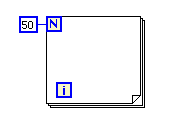In this for loop, the number 50 means that the code inside the FOR structure will be repeated 50 times, the iteration variable here is incremented +1 each iteration. The ‘i’ mark shows the iteration number starting from 0, 0-49 in this example. The equivalent code in C will be: for( i=0 ; i<50 ; i++ ){}

In onboard programming the FOR loop is quite different from this LabVIEW structure, the next picture will show the minimum code required: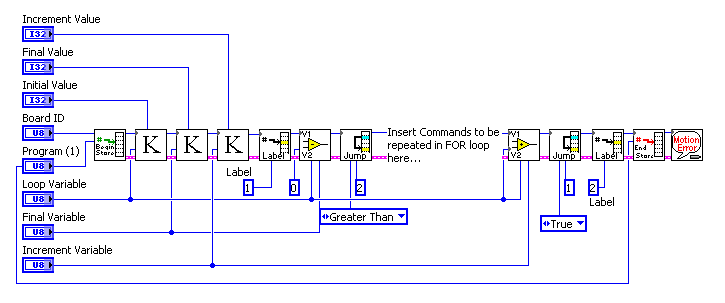Figure 1.

The way the previous code in the picture works is as follows, for the explanation we will use the same numbers (50 iterations) as the previous LabVIEW While For Loop picture: we need three variables in order to have a working FOR loop, the ‘loop variable’ which is the equivalent to ‘i’ in the LabVIEW or C example, and in the first ‘Load Constant To Variable’ function you initialize this ‘loop variable’ with a ‘initial value’, in this case 0, then you have a second variable called ‘final variable’ which will determine the maximum number for the ‘loop variable’ to have, in this case 49 (from 0 to 49 are 50 iterations); then you will load another variable called ‘increment variable’ with an ‘increment value’ of 1 for this example purposes, this variable will determine in which rate the ‘loop variable’ is increasing (or decreasing) its value’, it will be the same as i=i+1 or i++. After we initialize the variables we place a label number 1 on the code and we start checking the condition for the FOR loop to execute, if the ‘loop variable’ minus the ‘final variable’ (0 – 49) is greater than zero then jump to label 2, which is at the end of the loop structure and is for ‘exit’ or ‘break’ purposes; since at the beginning of the loop this comparison results in less than zero the Jump To Label On Condition function does not jumps to label 2, instead it executes the code that follows it. At the end of this code we have an Add Variables function to have the ‘loop variable’ increase its value +1, since we set the ‘increment variable’ to 1. Then we make a forced jump (Jump To Label On Condition set to TRUE) to label 1 which is at the beginning of the code to make the comparison again; now we have 1– 49 is still less than zero so the code executes one more time. The repetition continues until we have ‘loop variable’ with value 50, when the comparison is made 50 – 49 is ‘greater than’ zero as the condition is configured, so now we jump to label 2 which is the exit of the FOR loop.

The second loop structure we will explain is the DO-WHILE loop. It is equivalent to the LabVIEW While Loop structure that is shown below, we will make an example in which a loop will execute as until a variable reaches value zero (0) in order to exit the loop.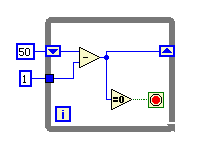Figure 2.

In this While loop we are executing the code inside it, which in this case is only a subtract operation and a zero comparison function a particular number of times defined by the condition. If you are familiar with LabVIEW this While loop is going to ‘exit’ once the shift registers value reaches zero. In onboard programming, the equivalent code is: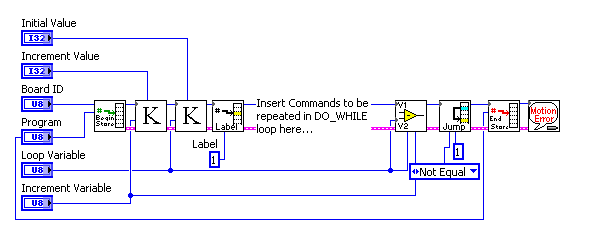Figure 3.

The previous code works exactly as the following C code:

do {
// code to be repeated
loopVar = loopVar - increment;
} while (loopVar != 0);

We begin the program storage and load two variables, one is the ‘loop variable’, which will have the value that defines the number of iterations on this case, we have then a second variable called ‘increment variable’ which will actually work as a decrement variable. We place a label number 1 and then the code that we want to be inside the loop. After the code ends we have a subtract operation, subtracting ‘loop variable’ minus the ‘increment variable’ and assigning that value back to ‘loop variable’, then we compare if its ‘not equal to zero’ and if its not then we will jump back to label 1 in order to have a loop structure. So on each iteration the value of ‘loop variable’ is changing and once it reaches zero it will not be ‘not equal’ to zero, which will cause the Jump On Condition function to skip the jump and just continue with the normal program flow on the next function. In this example the code is set as kind of a FOR loop, but depending whether how you program your algorithm you can make it undefined with an increment variable of zero, so the loop variable will not change its value, and inside the algorithm when your particular condition is meet change the value of the loop variable to zero so the subtract operation will be 0-0, equals zero, and the condition will not be met so it will exit instead of jumping back.

The most common condition structure known is the IF-ELSE. In LabVIEW the closest structure to it is the CASE box, in which you can have two different options and with a condition algorithm select either one.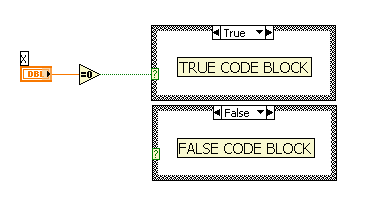Figure 4.

In this Case structure we are checking if the number (value) stored in variable ‘x’ is equal to zero, if it is then the TRUE code block executes, but if its not (else) the FALSE code block executes. In C you will have this code:

If ( x == 0 ){
// true code block
} else {
// false code block
}

If you would like to have the IF-ELSE structure in onboard you will code the following: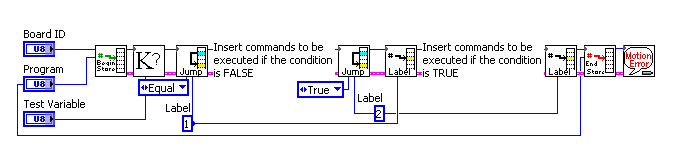Figure 5.

In this previous picture the code works by loading a ‘test variable’, if the value is ‘equal’ to zero (0) then jump to label 1 for which the code that follows that label is the ‘condition met’ or ‘true’ code block, but if the value was not zero then the code that follows the Jump On Condition function executes, which is mark as the ‘false’ code block, once the false code ends you have a Jump On Condition with condition = ‘true’ which means that you are forcing a jumping without any condition, so in this way you avoid executing the code that belongs to the ‘true’ code block. This is what makes an IF-ELSE statement in onboard programming.

As you can see with the loop and condition structures explained here, onboard programming is not as hard as it looks, since you can have a simple one-iteration flow program or you may make it more complex with loop and condition structures. This will help you in order to migrate your LabVIEW (or other language) program into an onboard code that will execute independently from your Windows Operating System.

In the next section we will review the basics of making arithmetic operations in onboard programming and special notes to take care of.

### Ratings

Rate this document

Answered Your Question?
Yes No